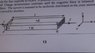# A Problem In Electromagnetic Phenomena

• vijayramakrishnan
In summary, the Hall effect is the tendency for electrons to accumulate on one side of a conductor due to a magnetic field. This results in an electric potential difference between the side with the electrons and the opposite side.

## Homework Statement

[/B]
In a thin rectangular metallic strip a constant current I flows along the positive x-direction, as shown in the figure. The length, width and thickness of the strip are l,w and d, respectively.

A uniform magnetic field B is applied on the strip along the positive y-direction. Due to this, the charge carriers experience a net deflection along the z-direction.

This results in accumulation of charge carriers on the surface PQRS and appearance of equal and opposite charges on the face opposite to PQRS. A potential difference along the z-direction is thus developed. Charge accumulation continues until the magnetic force is balanced by the electric force. The current is assumed to be uniformly distributed on the cross section of the strip and carried by electrons.

there is a charge accumulation on PQRS because of the force on the charge carriers but why should there be an opposite and equivalent charge induced on the face opposite to face PQRS

none

## The Attempt at a Solution

there is a charge accumulation on PQRS because of the force on the charge carriers but why should there be an opposite and equivalent charge induced on the face opposite to face PQRS

#### Attachments

•DSC_0474.JPG
29.2 KB · Views: 466
vijayramakrishnan said:
there is a charge accumulation on PQRS because of the force on the charge carriers but why should there be an opposite and equivalent charge induced on the face opposite to face PQRS.
Because when the valence electrons leave their atoms, the resulting atoms are now positively charged ions, of equal charge magnitude but opposite polarity.

BTW effect is called the Hall effect.

rude man said:
Because when the valence electrons leave their atoms, the resulting atoms are now positively charged ions, of equal charge magnitude but opposite polarity.

BTW effect is called the Hall effect.
sir,where are the valence electrons?,isn't it just charge caries so only electrons must be there

vijayramakrishnan said:
sir,where are the valence electrons?,isn't it just charge caries so only electrons must be there
sir i don't know anything about hall effect, this is a problem from high school syllabus only,we don't have any hall effect here,can it be solved through high school physics alone

There is no way to tell the difference between a positive charge and the absence of a negative charge.
Conversely, the absence of one sign of charge is the presence of the other sign of charge.
The point is that a potential difference is built up between PQRS and the opposite face.

vijayramakrishnan said:
sir,where are the valence electrons?,isn't it just charge caries so only electrons must be there
Based on the free-electron model in metals, electrons are the only moving charge carriers in a metal. The immobile positively charged ions are left behind on the side opposite to where the electrons are bunched. This creates an electric field which limits the bunching of the electrons. In the steady-state the E field and B field forces cancel each other. You need to determine the drift velocity vd of the electrons to determine numerically what the E field, and therefore the Hall voltage, are:
vd = I/neA ms-1
I = current, A
n = free-electron density, m-3 which varies from metal to metal.
e = 1.6e-19C
A = cross-section area of conductor.
Consult the Internet for the Hall effect.